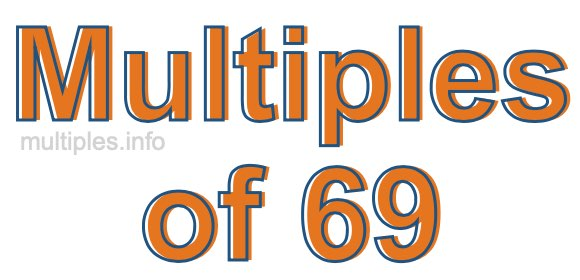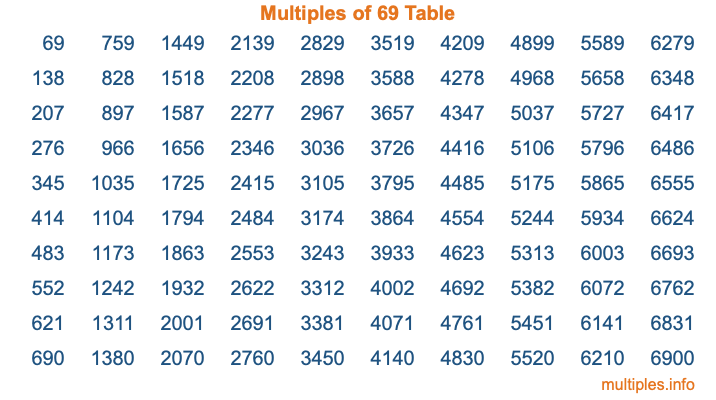Multiples of 69Welcome to the Multiples of 69 page. Here we will first teach you everything you will ever need to know about the multiples of 69, and then give you a study guide summary of everything we taught you to make sure you remember it all. Use this page to look up facts and learn information about the multiples of 69. This page will make you a multiples of sixty-nine expert!

Definition of Multiples of 69
Multiples of 69 are all the numbers that when divided by 69 equal an integer. Each of the multiples of 69 are called a multiple. A multiple of 69 is created by multiplying 69 by an integer.

Therefore, to create a list of multiples of 69, you start with 1 multiplied by 69, then 2 multiplied by 69, then 3 multiplied by 69, and so on for as long as you want. Thus, the list of the first five multiples of 69 is 69, 138, 207, 276, and 345. To see a larger list of multiples of 69, see the printable image of Multiples of 69 further down on this page. We also have a category where you can choose any nth multiple of 69.

Multiples of 69 Checker
The Multiples of 69 Checker below checks to see if any number of your choice is a multiple of 69. In other words, it checks to see if there is any number (integer) that when multiplied by 69 will equal your number. To do that, we divide your number by 69. If the the quotient is an integer, then your number is a multiple of 69.

Is  a multiple of 69?

Least Common Multiple of 69 and ...
A Least Common Multiple (LCM) is the lowest multiple that two or more numbers have in common. This is also called the smallest common multiple or lowest common multiple and is useful to know when you are adding our subtracting fractions. Enter one or more numbers below (69 is already entered) to find the LCM.

Check out our LCM Calculator if you need more details about the Least Common Multiple or if you need the LCM for different numbers for adding and subtraction fractions.

nth Multiple of 69
As we stated above, 69 is the first multiple of 69, 138 is the second multiple of 69, 207 is the third multiple of 69, and so on. Enter a number below to find the nth multiple of 69.

th multiple of 69

Multiples of 69 vs Factors of 69
69 is a multiple of 69 and a factor of 69, but that is where the similarities end. All postive multiples of 69 are 69 or greater than 69. All positive factors of 69 are 69 or less than 69.

Below is the beginning list of multiples of 69 and the factors of 69 so you can compare:

Multiples of 69: 69, 138, 207, 276, 345, etc.

Factors of 69: 1, 3, 23, 69

As you can see, the multiples of 69 are all the numbers that you can divide by 69 to get a whole number. The factors of 69, on the other hand, are all the whole numbers that you can multiply by another whole number to get 69.

It's also interesting to note that if a number (x) is a factor of 69, then 69 will also be a multiple of that number (x).

Multiples of 69 vs Divisors of 69
The divisors of 69 are all the integers that 69 can be divided by evenly. Below is a list of the divisors of 69.

Divisors of 69: 1, 3, 23, 69

The interesting thing to note here is that if you take any multiple of 69 and divide it by a divisor of 69, you will see that the quotient is an integer.

Multiples of 69 Table
Below is an image of the first 100 multiples of 69 in a table. The table is in chronological order, column by column. The first column has the first ten multiples of 69, the second column has the next ten multiples of 69, and so on.The Multiples of 69 Table is also referred to as the 69 Times Table or Times Table of 69. You are welcome to print out our table for your studies.

Negative Multiples of 69
Although not often discussed or needed in math, it is worth mentioning that you can make a list of negative multiples of 69 by multiplying 69 by -1, then by -2, then by -3, and so on, to get the following list of negative multiples of 69:

-69, -138, -207, -276, -345, etc.

Multiples of 69 Summary
Below is a summary of important Multiples of 69 facts that we have discussed on this page. To retain the knowledge on this page, we recommend that you read through the summary and explain to yourself or a study partner why they hold true.

There are an infinite number of multiples of 69.

A multiple of 69 divided by 69 will equal a whole number.

69 divided by a factor of 69 equals a divisor of 69.

The nth multiple of 69 is n times 69.

The largest factor of 69 is equal to the first positive multiple of 69.

69 is a multiple of every factor of 69.

69 is a multiple of 69.

A multiple of 69 divided by a divisor of 69 equals an integer.

69 divided by a divisor of 69 equals a factor of 69.

Any integer times 69 will equal a multiple of 69.

Multiples of a Number
Here you can get the multiples of another number, all with the same attention to detail as we did for multiples of 69 on this page.

Multiples of
Multiples of 70
Did you find our page about multiples of sixty-nine educational? Do you want more knowledge? Check out the multiples of the next number on our list!